Examples of the PlaneGeometry package

library(PlaneGeometry)

Exeter point

The Exeter point is defined as follows on Wikipedia.

Let $$ABC$$ be any given triangle. Let the medians through the vertices $$A$$, $$B$$, $$C$$ meet the circumcircle of triangle $$ABC$$ at $$A'$$, $$B'$$ and $$C'$$ respectively. Let $$DEF$$ be the triangle formed by the tangents at $$A$$, $$B$$, and $$C$$ to the circumcircle of triangle $$ABC$$. (Let $$D$$ be the vertex opposite to the side formed by the tangent at the vertex $$A$$, let $$E$$ be the vertex opposite to the side formed by the tangent at the vertex $$B$$, and let $$F$$ be the vertex opposite to the side formed by the tangent at the vertex $$C$$.) The lines through $$DA'$$, $$EB'$$ and $$FC'$$ are concurrent. The point of concurrence is the Exeter point of triangle $$ABC$$.

Let’s construct it with the PlaneGeometry package. We do not need to construct the triangle $$DEF$$: it is the tangential triangle of $$ABC$$, and is provided by the tangentialTriangle method of the R6 class Triangle.

A <- c(0,2); B <- c(5,4); C <- c(5,-1)
t <- Triangle$new(A, B, C) circumcircle <- t$circumcircle()
centroid <- t$centroid() medianA <- Line$new(A, centroid)
medianB <- Line$new(B, centroid) medianC <- Line$new(C, centroid)
Aprime <- intersectionCircleLine(circumcircle, medianA)[]
Bprime <- intersectionCircleLine(circumcircle, medianB)[]
Cprime <- intersectionCircleLine(circumcircle, medianC)[]
DEF <- t$tangentialTriangle() lineDAprime <- Line$new(DEF$A, Aprime) lineEBprime <- Line$new(DEF$B, Bprime) lineFCprime <- Line$new(DEF$C, Cprime) ( ExeterPoint <- intersectionLineLine(lineDAprime, lineEBprime) ) #>  2.621359 1.158114 # check whether the Exeter point is also on (FC') lineFCprime$includes(ExeterPoint)
#>  TRUE

Let’s draw a figure now.

opar <- par(mar = c(0,0,0,0))
plot(NULL, asp = 1, xlim = c(-2,9), ylim = c(-6,7),
xlab = NA, ylab = NA, axes = FALSE)
draw(t, lwd = 2, col = "black")
draw(circumcircle, lwd = 2, border = "cyan")
draw(Triangle$new(Aprime,Bprime,Cprime), lwd = 2, col = "green") draw(DEF, lwd = 2, col = "blue") draw(Line$new(ExeterPoint, DEF$A, FALSE, FALSE), lwd = 2, col = "red") draw(Line$new(ExeterPoint, DEF$B, FALSE, FALSE), lwd = 2, col = "red") draw(Line$new(ExeterPoint, DEF$C, FALSE, FALSE), lwd = 2, col = "red") points(rbind(ExeterPoint), pch = 19, col = "red")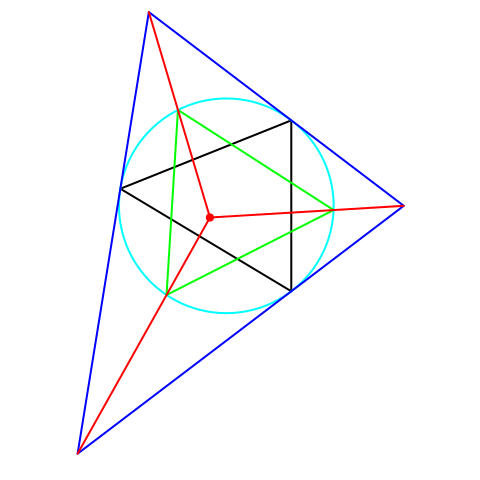par(opar) Circles tangent to three circles Let $$\mathcal{C}_1$$, $$\mathcal{C}_2$$ and $$\mathcal{C}_3$$ be three circles with respective radii $$r_1$$, $$r_2$$ and $$r_3$$ such that $$r_3 < r_1$$ and $$r_3 < r_2$$. How to construct some circles simultaneously tangent to these three circles? C1 <- Circle$new(c(0,0), 2)
C2 <- Circle$new(c(5,5), 3) C3 <- Circle$new(c(6,-2), 1)
# inversion swapping C1 and C3 with positive power
iota1 <- inversionSwappingTwoCircles(C1, C3, positive = TRUE)
# inversion swapping C2 and C3 with positive power
iota2 <- inversionSwappingTwoCircles(C2, C3, positive = TRUE)
# take an arbitrary point on C3
M <- C3$pointFromAngle(0) # invert it with iota1 and iota2 M1 <- iota1$invert(M); M2 <- iota2$invert(M) # take the circle C passing through M, M1, M2 C <- Triangle$new(M,M1,M2)$circumcircle() # take the line passing through the two inversion poles cl <- Line$new(iota1$pole, iota2$pole)
# take the radical axis of C and C3
L <- C$radicalAxis(C3) # let H bet the intersection of these two lines H <- intersectionLineLine(L, cl) # take the circle Cp with diameter [HO3] O3 <- C3$center
Cp <- CircleAB(H, O3)
# get the two intersection points T0 and T1 of C3 with Cp
T0_and_T1 <- intersectionCircleCircle(C3, Cp)
T0 <- T0_and_T1[[1L]]; T1 <- T0_and_T1[[2L]]
# invert T0 with respect to the two inversions
T0p <- iota1$invert(T0); T0pp <- iota2$invert(T0)
# the circle passing through T0 and its two images is a solution
Csolution0 <- Triangle$new(T0, T0p, T0pp)$circumcircle()
# invert T1 with respect to the two inversions
T1p <- iota1$invert(T1); T1pp <- iota2$invert(T1)
# the circle passing through T1 and its two images is another solution
Csolution1 <- Triangle$new(T1, T1p, T1pp)$circumcircle()
opar <- par(mar = c(0,0,0,0))
plot(NULL, asp = 1, xlim = c(-4,9), ylim = c(-4,9),
xlab = NA, ylab = NA, axes = FALSE)
draw(C1, col = "yellow", border = "red")
draw(C2, col = "yellow", border = "red")
draw(C3, col = "yellow", border = "red")
draw(Csolution0, lwd = 2, border = "blue")
draw(Csolution1, lwd = 2, border = "blue")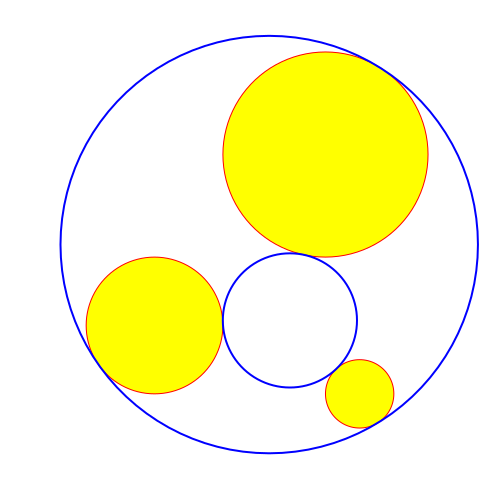par(opar)

Apollonius circle of a triangle

There are several circles called “Apollonius circle”. We take the one defined as follows, with respect to a reference triangle: the circle which touches all three excircles of the reference triangle and encompasses them.

It can be constructed as the inversive image of the nine-point circle with respect to the circle orthogonal to the excircles of the reference triangle. This inversion can be obtained in PlaneGeometry with the function inversionFixingThreeCircles.

# reference triangle
t <- Triangle$new(c(0,0), c(5,3), c(3,-1)) # nine-point circle npc <- t$orthicTriangle()$circumcircle() # excircles excircles <- t$excircles()
# inversion with respect to the circle orthogonal to the excircles
iota <- inversionFixingThreeCircles(excircles$A, excircles$B, excircles$C) # Apollonius circle ApolloniusCircle <- iota$invertCircle(npc)

Let’s do a figure:

opar <- par(mar = c(0,0,0,0))
plot(NULL, asp = 1, xlim = c(-10,14), ylim = c(-5, 18),
xlab = NA, ylab = NA, axes = FALSE)
draw(t, lwd = 2)
draw(excircles$A, lwd = 2, border = "blue") draw(excircles$B, lwd = 2, border = "blue")
draw(excircles$C, lwd = 2, border = "blue") draw(ApolloniusCircle, lwd = 2, border = "red")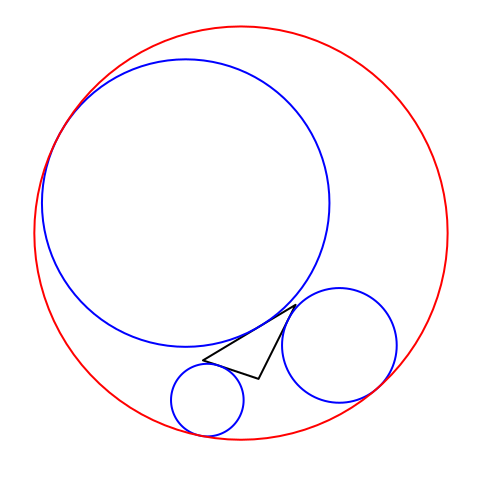par(opar) The radius of the Apollonius circle is $$\frac{r^2+s^2}{4r}$$ where $$r$$ is the inradius of the triangle and $$s$$ its semiperimeter. Let’s check this point: inradius <- t$inradius()
semiperimeter <- sum(t$edges()) / 2 (inradius^2 + semiperimeter^2) / (4*inradius) #>  11.15942 ApolloniusCircle$radius
#>  11.15942

Filling the lapping area of two circles

Let two circles intersecting at two points. How to fill the lapping area of the two circles?

O1 <- c(2,5); circ1 <- Circle$new(O1, 2) O2 <- c(4,4); circ2 <- Circle$new(O2, 3)

opar <- par(mar = c(0,0,0,0))
plot(NULL, asp = 1, xlim = c(0,8), ylim = c(0,8), xlab = NA, ylab = NA)
draw(circ1, border = "purple", lwd = 2)
draw(circ2, border = "forestgreen", lwd = 2)

intersections <- intersectionCircleCircle(circ1, circ2)
A <- intersections[]; B <- intersections[]
points(rbind(A,B), pch = 19, col = c("red", "blue"))

theta1 <- Arg((A-O1) + 1i*(A-O1))
theta2 <- Arg((B-O1) + 1i*(B-O1))
path1 <- Arc$new(O1, circ1$radius, theta1, theta2, FALSE)$path() theta1 <- Arg((A-O2) + 1i*(A-O2)) theta2 <- Arg((B-O2) + 1i*(B-O2)) path2 <- Arc$new(O2, circ2$radius, theta2, theta1, FALSE)$path()

polypath(rbind(path1,path2), col = "yellow")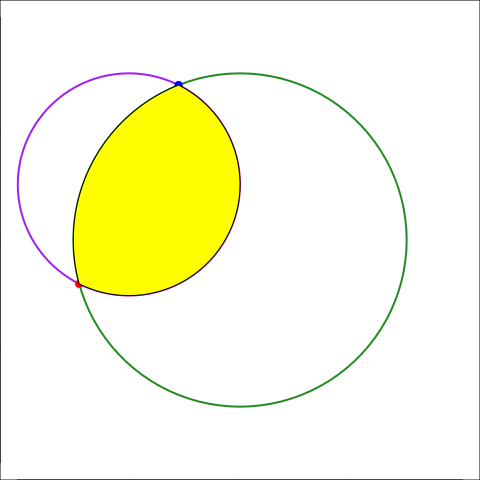par(opar)

Hyperbolic tessellation

In the help page of the Circle R6 class (?Circle), we show how to draw a hyperbolic triangle with the help of the method orthogonalThroughTwoPointsOnCircle(). Here we will use this method to draw a hyperbolic tessellation.

tessellation <- function(depth, Thetas0, colors){
stopifnot(
depth >= 3,
is.numeric(Thetas0),
length(Thetas0) == 3L,
is.character(colors),
length(colors) >= depth
)

circ <- Circle$new(c(0,0), 3) arcs <- lapply(seq_along(Thetas0), function(i){ ip1 <- ifelse(i == length(Thetas0), 1L, i+1L) circ$orthogonalThroughTwoPointsOnCircle(Thetas0[i], Thetas0[ip1],
arc = TRUE)
})
inversions <- lapply(arcs, function(arc){
Inversion$new(arc$center, arc$radius^2) }) Ms <- vector("list", depth) Ms[[1L]] <- lapply(Thetas0, function(theta) c(cos(theta), sin(theta))) Ms[[2L]] <- vector("list", 3L) for(i in 1L:3L){ im1 <- ifelse(i == 1L, 3L, i-1L) M <- inversions[[i]]$invert(Ms[[1L]][[im1]])
attr(M, "iota") <- i
Ms[[2L]][[i]] <- M
}

for(d in 3L:depth){
n1 <- length(Ms[[d-1L]])
n2 <- 2L*n1
Ms[[d]] <- vector("list", n2)
k <- 0L
while(k < n2){
for(j in 1L:n1){
M <- Ms[[d-1L]][[j]]
for(i in 1L:3L){
if(i != attr(M, "iota")){
k <- k + 1L
newM <- inversions[[i]]$invert(M) attr(newM, "iota") <- i Ms[[d]][[k]] <- newM } } } } } # plot #### opar <- par(mar = c(0,0,0,0), bg = "black") plot(NULL, asp = 1, xlim = c(-4,4), ylim = c(-4,4), xlab = NA, ylab = NA, axes = FALSE) draw(circ, border = "white") invisible(lapply(arcs, draw, col = colors[1L], lwd = 2)) Thetas <- lapply( rapply(Ms, function(M){ Arg(M[1L] + 1i*M[2L]) }, how="replace"), unlist) for(d in 2L:depth){ thetas <- sort(unlist(Thetas[1L:d])) for(i in 1L:length(thetas)){ ip1 <- ifelse(i == length(thetas), 1L, i+1L) arc <- circ$orthogonalThroughTwoPointsOnCircle(thetas[i], thetas[ip1],
arc = TRUE)
draw(arc, lwd = 2, col = colors[d])
}
}

par(opar)

invisible()
}
tessellation(
depth = 5L,
Thetas0 = c(0, 2, 3.8),
colors = viridisLite::viridis(5)
)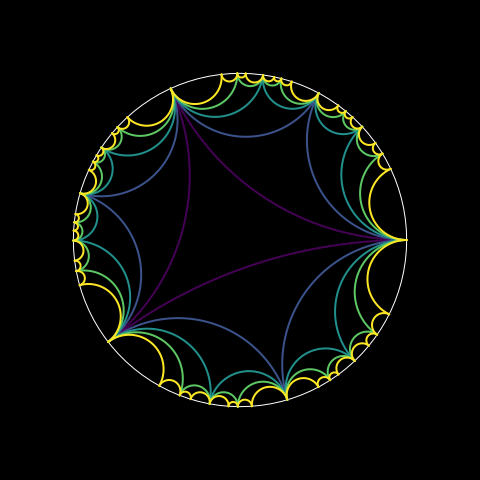Here is a version which allows to fill the hyperbolic triangles:

tessellation2 <- function(depth, Thetas0, colors){
stopifnot(
depth >= 3,
is.numeric(Thetas0),
length(Thetas0) == 3L,
is.character(colors),
length(colors)-1L >= depth
)

circ <- Circle$new(c(0,0), 3) arcs <- lapply(seq_along(Thetas0), function(i){ ip1 <- ifelse(i == length(Thetas0), 1L, i+1L) circ$orthogonalThroughTwoPointsOnCircle(Thetas0[i], Thetas0[ip1],
arc = TRUE)
})
inversions <- lapply(arcs, function(arc){
Inversion$new(arc$center, arc$radius^2) }) Ms <- vector("list", depth) Ms[[1L]] <- lapply(Thetas0, function(theta) c(cos(theta), sin(theta))) Ms[[2L]] <- vector("list", 3L) for(i in 1L:3L){ im1 <- ifelse(i == 1L, 3L, i-1L) M <- inversions[[i]]$invert(Ms[[1L]][[im1]])
attr(M, "iota") <- i
Ms[[2L]][[i]] <- M
}

for(d in 3L:depth){
n1 <- length(Ms[[d-1L]])
n2 <- 2L*n1
Ms[[d]] <- vector("list", n2)
k <- 0L
while(k < n2){
for(j in 1L:n1){
M <- Ms[[d-1L]][[j]]
for(i in 1L:3L){
if(i != attr(M, "iota")){
k <- k + 1L
newM <- inversions[[i]]$invert(M) attr(newM, "iota") <- i Ms[[d]][[k]] <- newM } } } } } # plot #### opar <- par(mar = c(0,0,0,0), bg = "black") plot(NULL, asp = 1, xlim = c(-4,4), ylim = c(-4,4), xlab = NA, ylab = NA, axes = FALSE) path <- do.call(rbind, lapply(rev(arcs), function(arc) arc$path()))
polypath(path, col = colors[1L])

invisible(lapply(arcs, function(arc){
path1 <- arc$path() B <- arc$startingPoint()
A <- arc$endingPoint() alpha1 <- Arg(A[1L] + 1i*A[2L]) alpha2 <- Arg(B[1L] + 1i*B[2L]) path2 <- Arc$new(c(0,0), 3, alpha1, alpha2, FALSE)$path() polypath(rbind(path1,path2), col = colors[2L]) })) Thetas <- lapply( rapply(Ms, function(M){ Arg(M[1L] + 1i*M[2L]) }, how="replace"), unlist) for(d in 2L:depth){ thetas <- sort(unlist(Thetas[1L:d])) for(i in 1L:length(thetas)){ ip1 <- ifelse(i == length(thetas), 1L, i+1L) arc <- circ$orthogonalThroughTwoPointsOnCircle(thetas[i], thetas[ip1],
arc = TRUE)
path1 <- arc$path() B <- arc$startingPoint()
A <- arc$endingPoint() alpha1 <- Arg(A[1L] + 1i*A[2L]) alpha2 <- Arg(B[1L] + 1i*B[2L]) path2 <- Arc$new(c(0,0), 3, alpha1, alpha2, FALSE)$path() polypath(rbind(path1,path2), col = colors[d+1L]) } } draw(circ, border = "white") par(opar) invisible() } tessellation2( depth = 5L, Thetas0 = c(0, 2, 3.8), colors = viridisLite::viridis(6) )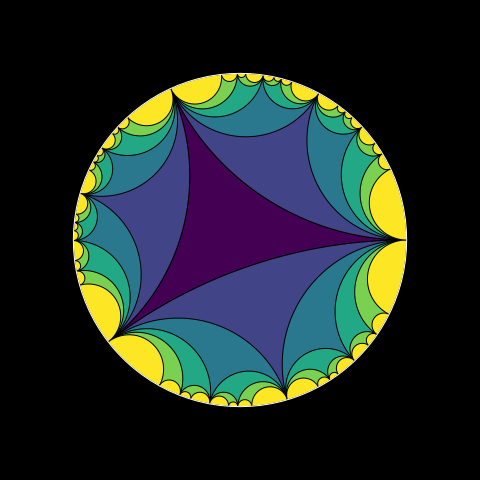Director circle of an ellipse Let’s draw the director circle of an ellipse. We start by constructing the minimum bounding box of the ellipse. ell <- Ellipse$new(c(1,1), 5, 2, 30)
majorAxis <- ell$diameter(0) minorAxis <- ell$diameter(pi/2)
v1 <- (majorAxis$B - majorAxis$A) / 2
v2 <- (minorAxis$B - minorAxis$A) / 2
# sides of the minimum bounding box
side1 <- majorAxis$translate(v2) side2 <- majorAxis$translate(-v2)
side3 <- minorAxis$translate(v1) side4 <- minorAxis$translate(-v1)
# take a vertex of the bounding box
A <- side1$A # director circle circ <- CircleOA(ell$center, A)

Now let’s take a tangent $$T_1$$ to the ellipse, construct the half-line directed by $$T_1$$ with origin the point of tangency, determine the intersection point of this half-line with the director circle, and draw the perpendicular $$T_2$$ of $$T_1$$ passing by this intersection point. Then $$T_2$$ is another tangent to the ellipse.

T1 <- ell$tangent(0.3) halfT1 <- T1$clone(deep = TRUE)
halfT1$extendA <- FALSE I <- intersectionCircleLine(circ, halfT1, strict = TRUE) T2 <- T1$perpendicular(I)
opar <- par(mar=c(0,0,0,0))
plot(NULL, asp = 1,
xlim = c(-3,6), ylim = c(-5,7), xlab = NA, ylab = NA)
# draw the ellipse
draw(ell, col = "blue")
# draw the bounding box
draw(side1, lwd = 2, col = "green")
draw(side2, lwd = 2, col = "green")
draw(side3, lwd = 2, col = "green")
draw(side4, lwd = 2, col = "green")
# draw the director circle
draw(circ, lwd = 2, border = "red")
# draw the two tangents
draw(T1); draw(T2)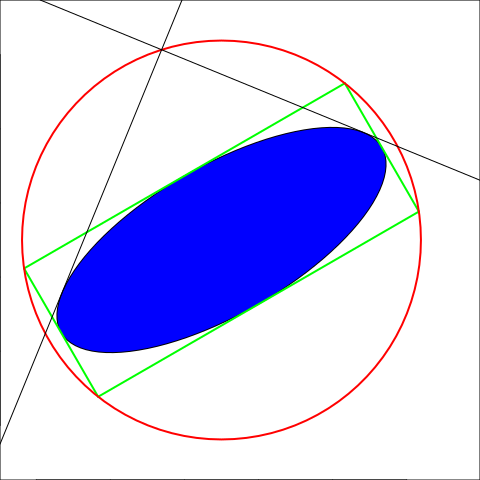# restore the graphical parameters
par(opar)

Playing with Steiner chains

The PlaneGeometry package has a function SteinerChain which generates a Steiner chain of circles.

Elliptical Steiner chain

By applying an affine transformation to a Steiner chain, we can get an elliptical Steiner chain.

c0 <- Circle$new(c(3,0), 3) # exterior circle circles <- SteinerChain(c0, 3, -0.2, 0.5) # take an ellipse ell <- Ellipse$new(c(-4,0), 4, 2.5, 140)
# take the affine transformation which maps the exterior circle to this ellipse
f <- AffineMappingEllipse2Ellipse(c0, ell)
# take the images of the Steiner circles by this transformation
ellipses <- lapply(circles, f$transformEllipse) opar <- par(mar = c(0,0,0,0)) plot(NULL, asp = 1, xlim = c(-8,6), ylim = c(-4,4), xlab = NA, ylab = NA, axes = FALSE) # draw the Steiner chain invisible(lapply(circles, draw, lwd = 2, col = "blue")) draw(c0, lwd = 2) # draw the elliptical Steiner chain invisible(lapply(ellipses, draw, lwd = 2, col = "red", border = "forestgreen")) draw(ell, lwd = 2, border = "forestgreen")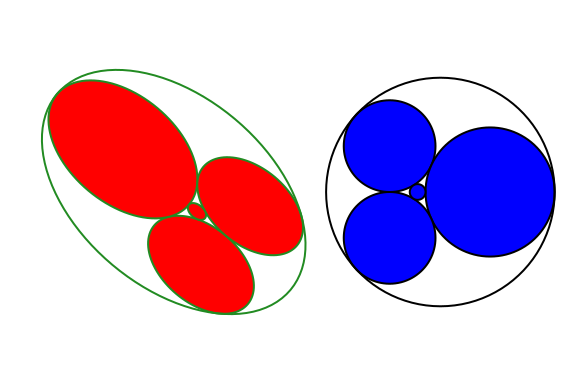par(opar) Here is how I got the animation below, by varying the shift parameter of the Steiner chain. library(gifski) c0 <- Circle$new(c(3,0), 3)
ell <- Ellipse$new(c(-4,0), 4, 2.5, 140) f <- AffineMappingEllipse2Ellipse(c0, ell) fplot <- function(shift){ circles <- SteinerChain(c0, 3, -0.2, shift) ellipses <- lapply(circles, f$transformEllipse)
opar <- par(mar = c(0,0,0,0))
plot(NULL, asp = 1, xlim = c(-8,0), ylim = c(-4,4),
xlab = NA, ylab = NA, axes = FALSE)
invisible(lapply(ellipses, draw, lwd = 2, col = "blue", border = "black"))
draw(ell, lwd = 2)
par(opar)
invisible()
}

shift_ <- seq(0, 3, length.out = 100)[-1L]

save_gif(
for(shift in shift_){
fplot(shift)
},
"SteinerChainElliptical.gif",
512, 512, 1/12, res = 144
)